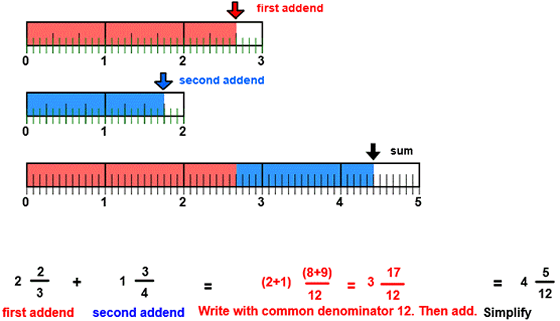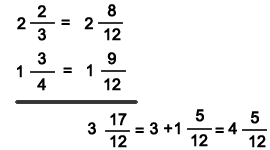CORRECT:
ATTEMPTS:
SCORE:
PERCENT
WHOLE
NUMERATOR
DENOMINATOR

# ADD UNLIKE MIXED FRACTIONS INSTRUCTION

Follow the directions in the dialog box after pressing the <START> button. The <EXPLAIN> button may be pressed after you enter the second addend to see how to do the example.

When the program starts, you will be asked to identify the first addend and then the second addend. The program will not continue unless each addend is correctly identified. You will then be asked to add each addend for the sum.Addition with Fractions - Simplify is similar to the previous program ADD UNLIKE FRACTIONS except that the addends may be mixed numbers and the sum must be entered so that the numerator is smaller than the denominator and the numerator and denominator are in lowest terms.

See the program MIXED NUMBERS for information on writing fractions in mixed form.

See the program RENAME IN LOWEST TERMS for information on writing fractions in lowest terms.

The above image shows a first addend of 2 23 in red and a second addend of 1 34 in blue. The least common denominator of 3 and 4 is 12 and is shown in both addends. So the first addend 2 23 also shows 2 812 and the second addend 1 34 also shows 1 912 .

Now that both addends are written with a common denominator the numerators can be combined for the sum. This is shown in the sum image. Notice how in the sum image that the first addend in red and the second addend in blue are combined for a sum of 4 512 .

The math section of the illustration shows that the addends are written with a common denominator 12. The numerators 8 and 9 are then added for a numerator of 17 in the sum. The 1712 in the sum can be simplified to 1 512 in mixed form. The 1 in 1 512 is added to 3 for a simplified sum of 4 512. The sum must be entered in lowest terms. Although 3 1712 is correct only 4 512 is acceptable for the simplest form of the sum.

Another method is to work vertically as shown below:In many examples, the sum may be found by visually combining the two addends.

In the above example, the sum (4 512) must be entered as a mixed number and in lowest terms.

For more instruction on adding fractions go to How To Add Fractions.

After you enter the sum you may press the <REPORT> button. The report will ask for your name but you may submit a code for your name. This report will give the same results as on the dialog box. The report may be printed or e-mailed.